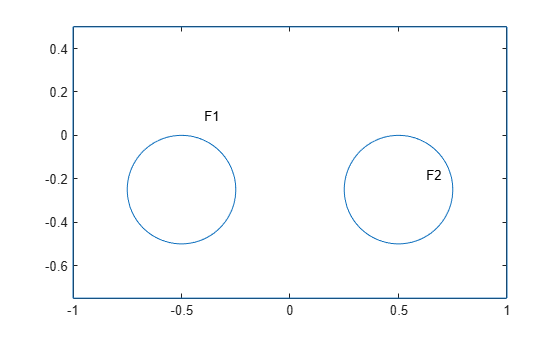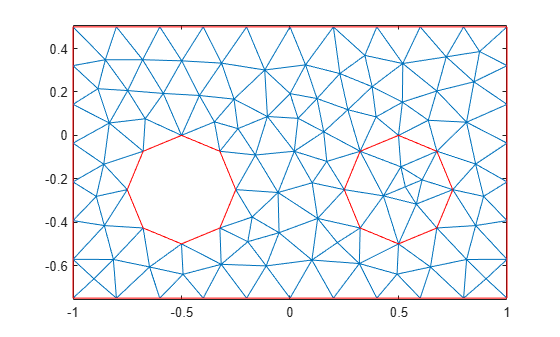# pdesde

Indices of edges of mesh elements belonging to the specified faces of 2-D geometry

`pdesde` is not recommended. Use `faceEdges` instead.

## Syntax

``i = pdesde(e,FaceID)``
``i = pdesde(e)``

## Description

example

````i = pdesde(e,FaceID)` returns the indices of the `[p,e,t]` mesh edges that belong to outer boundaries of the geometry for a set of faces listed in `FaceID`.```
````i = pdesde(e)` assumes that `FaceID` is a list of all faces of a 2-D geometry.```

## Examples

collapse all

Find the indices of the mesh elements' edges located on the outer boundaries of the geometry and belonging to the specified faces.

Define two circles and a rectangle and place these in one matrix.

```R1 = [3,4,-1,1,1,-1,0.5,0.5,-0.75,-0.75]'; C1 = [1,-0.5,-0.25,0.25]'; C2 = [1,0.5,-0.25,0.25]'; C1 = [C1;zeros(length(R1) - length(C1),1)]; C2 = [C2;zeros(length(R1) - length(C2),1)]; gd = [R1,C1,C2];```

Create a set formula that subtracts one circle from the rectangle and adds the other circle to the rectangle.

`sf = 'R1-C1+C2';`

Create and plot the geometry.

```ns = char('R1','C1','C2'); ns = ns'; gd = decsg(gd,sf,ns); pdegplot(gd,'FaceLabels','on')```Create and plot a mesh.

```[p,e,t] = initmesh(gd); pdemesh(p,e,t)```Find the indices of the mesh elements' edges located on the outer boundaries and belonging to face 1. Display the result as a column vector.

```i1 = pdesde(e,1); i1.'```
```ans = 42×1 1 2 3 4 5 6 7 8 9 10 ⋮ ```

The resulting vector contains indices of all mesh edges in this geometry, except the eight internal edges surrounding face 2.

`length(e) - length(i1)`
```ans = 8 ```

Use the `pdesde` function to find the mesh edges surrounding face 2. The result is an empty vector because none of these mesh edges belong to the outer boundary of the geometry.

`i2 = pdesde(e,2)`
```i2 = 1x0 empty double row vector ```

## Input Arguments

collapse all

Mesh edges, specified as a `7`-by-`Ne` matrix of edges, where `Ne` is the number of edges in the mesh. For details on the mesh data representation, see `initmesh`.

Data Types: `double`

Face IDs, specified as a vector of integers.

Data Types: `double`

## Output Arguments

collapse all

Indices of the mesh edges on the outer boundaries, returned as a vector of integers.

### Topics

Introduced before R2006a

## SupportGet trial now